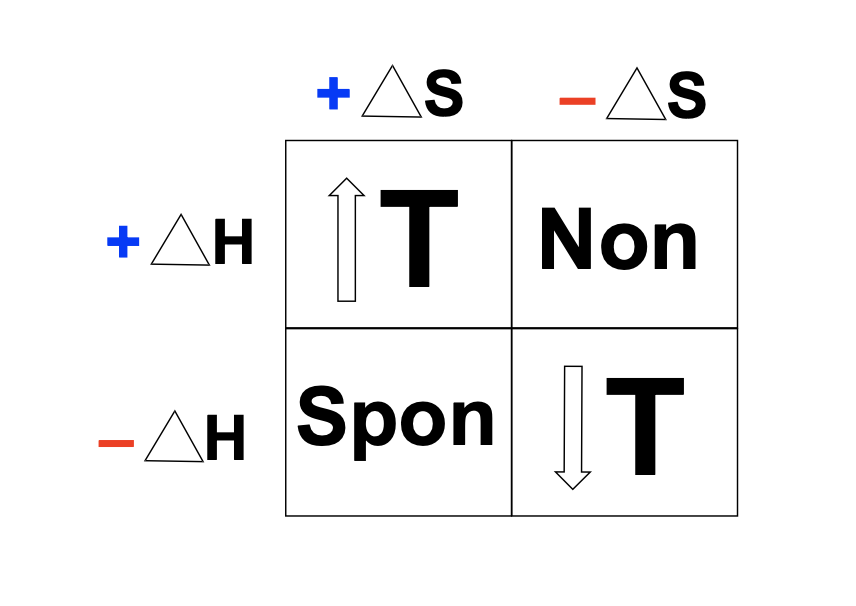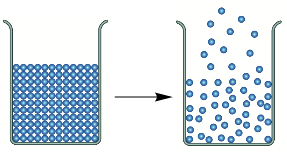Clutch Prep is now a part of Pearson
Ch.17 - Chemical ThermodynamicsWorksheetSee all chapters

# Gibbs Free Energy

See all sections
Sections
Spontaneous vs Nonspontaneous Reactions
Entropy
Entropy Calculations
Entropy Calculations: Phase Changes
Third Law of Thermodynamics
Gibbs Free Energy
Gibbs Free Energy Calculations
Gibbs Free Energy And Equilibrium

Gibbs Free Energy represents the energy associated with a chemical reaction that can be utilized to do work.

###### Understanding Gibbs Free Energy

Concept #1: By examining either Gibbs Free Energy or the Equilibrium constant the spontaneity of a chemical reaction can be determined.

Example #1: If ∆G is small and positive, which of the following statements is true?

a) the forward reaction is spontaneous and system is far from equilibrium

b) the forward reaction is spontaneous and system is near equilibrium

c) the reverse reaction is spontaneous and system is far from equilibrium

d) the reverse reaction is spontaneous and system is near equilibrium

Concept #2: Different temperature conditions can lead to an increase, decrease or no change in spontaneity:Practice: What are the signs of ∆H, ∆S and ∆G for the spontaneous conversion of a solid into gas?Practice: The chemical reaction 2 NO2Br (g) → 2 NO2 (g) + Br2 (g) has a Keq = 4.50 × 105.

Does the reaction increase the entropy of the Universe? Explain.

Example #2: PCl3 (g) + Cl2 (g) ⇌ PCl5 (g)  At 25°C, ΔH° = - 92.50 kJ. Which of the following statements is(are) true?

a. This is an endothermic reaction.

b. If the temperature is increased, the ratio of [PCl5] / [PCl3] [Cl2] will increase.

c. ΔS° for this reaction is negative.

d. ΔG° for this reaction has to be negative at all temperatures.

Practice: Consider the combustion of butane gas and predict the signs of ΔS, ΔH and ∆G.

C4H10 (g) + 13/2 O2 (g)  ⟶  4 CO2 (g) + 5 H2O (g)

Practice: You calculate the value of ΔG for a chemical reaction and get a positive value. Which would be the most accurate way to interpret this result?

a) If a mixture of reactants and products is created and left to equilibrate, the equilibrium mixture will contain more reactant than product.

b) If a mixture of reactants and products is created, we cannot say anything about its composition at equilibrium but we can say it will reach equilibrium very rapidly.

c) The reaction will not occur under any circumstances.

d) If a mixture of reactants and products is created and left to equilibrate, the equilibrium mixture will contain more product than reactant.